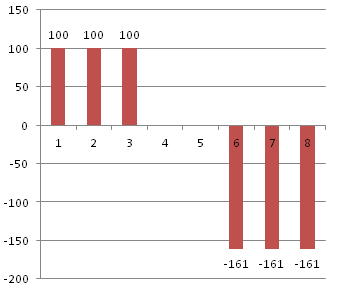A series of cash flows over a span of 8 years have a present value of 0. The series consist of a...

Question:

A series of cash flows over a span of 8 years have a present value of 0. The series consist of a positive disbursement of $100 at years 1, 2, and 3, and a negative disbursement of$A at years 6, 7, and 8. Compute the value of disbursement \$A, assuming a 10% interest rate. Draw the cash flow diagram.

Cash Flow Diagram:

A cash flow diagram of a graphical representation of cash flows. The diagram shows the timing, direction (outflow or inflow) and amount of each payment in a cash flow.

The value of the disbursement is such that the value of the negative disbursement, measured at the end of year 3, is equal to the value of the positive disbursements at that time. We can use the following formula to compute the present value of an annuity with periodic payment {eq}M {/eq} for {eq}T{/eq} periods, given periodic return {eq}r{/eq}:

• {eq}\displaystyle \frac{M(1 - (1 + r)^{-T})}{r} {/eq}

We can use the following formula to compute the future value of an annuity with periodic payment {eq}M {/eq} for {eq}T{/eq} periods, given periodic return {eq}r{/eq}:

• {eq}\displaystyle \frac{M((1 + r)^T - 1)}{r} {/eq}

Applying the formulas, we have:

• {eq}\displaystyle \frac{100*((1 + 10\%)^3 - 1)}{10\%} = -\displaystyle \frac{A*(1 - (1 + 10\%)^{-3})}{10\%(1 + 10\%)^2} {/eq}
• {eq}331 = -2.055249579 * A {/eq}
• {eq}A = -161.051 {/eq}

The cash flow diagram is plotted below: